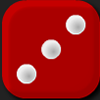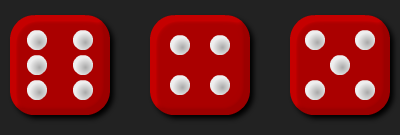#### You may also like### Round the Four Dice

This activity involves rounding four-digit numbers to the nearest thousand.### Round the Dice Decimals 2

What happens when you round these numbers to the nearest whole number?### Round the Dice Decimals 1

Use two dice to generate two numbers with one decimal place. What happens when you round these numbers to the nearest whole number?

# Round the Three Dice

##### Age 7 to 11Challenge LevelThere are three dice, each of them with faces labelled from 1 to 6.
When the dice are rolled they can be combined in six different ways to make a three-digit number.

For example, if I roll a 2 and a 4 and a 5, I can combine them to make 245, 254, 425, 452, 524 or 542.

Now round each of these numbers to the nearest 100:
245 rounds to 200, 254 rounds to 300, 425 rounds to 400, 452 rounds to 500, 524 rounds to 500 and 542 rounds to 500.

Repeat for other rolls of the dice.

Can each of the six numbers round to the same multiple of 100?
Can each of the six numbers round to a different multiple of 100?

There some interactive dice here that you can use for this problem.